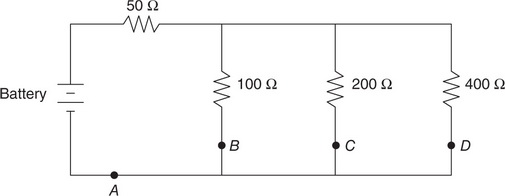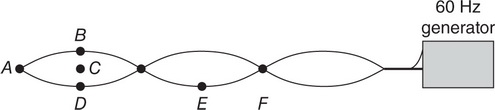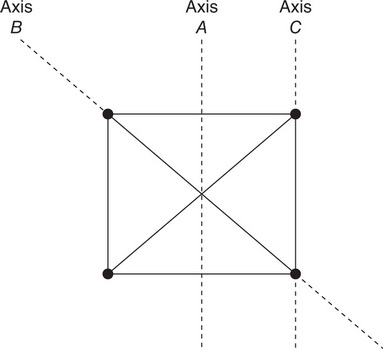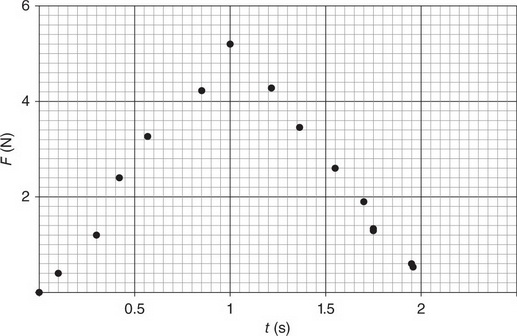# AP Physics 1 Practice Test 11

### Test Information10 questions18 minutes

1. A circuit consists of a battery and a light bulb. At first, the circuit is disconnected. Then, the circuit is connected, and the light bulb lights. After the light bulb has been lit for a few moments, how has the net charge residing on the circuit elements changed?

2.At which position in the above circuit will the charge passing that position in one second be largest?

3. Spring scales are used to measure the net force applied to an object; a sonic motion detector is used to measure the object’s resulting acceleration. A graph is constructed with the net force on the vertical axis and the acceleration on the horizontal axis. Which of the following quantities is directly measured using the slope of this graph?

4.In the laboratory, a 0.5-kg cart collides with a fixed wall, as shown in the preceding diagram. The collision is recorded with a video camera that takes 20 frames per second. A student analyzes the video, placing a dot at the center of mass of the cart in each frame. The analysis is shown above.

About how fast was the cart moving before the collision?

5.In the laboratory, a 0.5-kg cart collides with a fixed wall, as shown in the preceding diagram. The collision is recorded with a video camera that takes 20 frames per second. A student analyzes the video, placing a dot at the center of mass of the cart in each frame. The analysis is shown above.

Which of the following best estimates the change in the cart’s momentum during the collision?

6.In the laboratory, a 60-Hz generator is connected to a string that is fixed at both ends. A standing wave is produced, as shown in the preceding figure. In order to measure the wavelength of this wave, a student should use a meterstick to measure from positions

7.Four identical lead balls with large mass are connected by rigid but very light rods in the square configuration shown in the preceding figure. The balls are rotated about the three labeled axes. Which of the following correctly ranks the rotational inertia I of the balls about each axis?

8. In the laboratory, a cart experiences a single horizontal force as it moves horizontally in a straight line. Of the following data collected about this experiment, which is sufficient to determine the work done on the cart by the horizontal force?

9.A wave pulse on a string is shown above. Which pulse, when superimposed with the one above, will produce complete destructive interference?

10.In the laboratory, a 3-kg cart experiences a varying net force. This net force is measured as a function of time, and the data collected are displayed in the graph above. What is the change in the cart’s momentum during the interval t = 0 to t = 2 s?